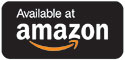Catalog Higher Ed Introduction to Differential Equations 2e

# Introduction to Differential Equations 2e

## by Mark H. Holmes

Second Edition

### Print Version

#### Paperback Price: \$53.57

ISBN: 978-1-97507-720-4

239 pages

Suggested retail price shown above.This textbook, in its second edition, is a concise introduction to differential equations for students in science and engineering. Emphasis is placed on the methods of solution, on the constructive use of qualitative analysis, and on the interconnections between differential equations and mathematical modeling. It is written in an accessible, easy-to-read, format. There are numerous worked out examples and exercises in each section, along with a wide variety of applications. The text has also been written with affordability and flexibility in mind. The author Mark H Holmes Ph.D. is an applied mathematician who has written several successful undergraduate and graduate textbooks on mathematical modeling and scientific computing.

This textbook was written as an introductory course in differential equations. While often taught in mathematics departments, it is not uncommon that this topic is also found in engineering departments or other school departments. The expectation is that the students will have taken at least a year of calculus before approaching this subject.

Mark H. Holmes Ph.D. works in the Department of Mathematical Sciences Rensselaer Polytechnic Institute, Troy, NY.